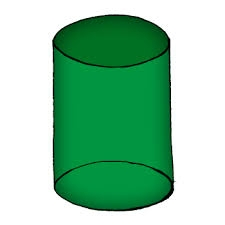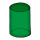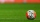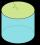# I need

I need to calculate the height of the cylinder. I have a given that the radius is 6 cm and the volume is 282.6 cm3. What is the formula for this?

h =  2.4987 cm

### Step-by-step explanation:Did you find an error or inaccuracy? Feel free to write us. Thank you!#### You need to know the following knowledge to solve this word math problem:

We encourage you to watch this tutorial video on this math problem:

## Related math problems and questions:

• Cylinder - hThe cylinder volume is 140 cm3. The base radius is 7 cm. Calculate the height of the cylinder.
• Height of the cylinderThe cylinder volume is 150 dm cubic, the base diameter is 100 cm. What is the height of the cylinder?
• Diameter of a cylinderI need to calculate the cylinder volume with a height of 50 cm and a diameter of 30 cm.
• The cylinderIn a rotating cylinder it is given: the surface of the shell (without bases) S = 96 cm2 and the volume V = 192 cm cubic. Calculate the radius and height of this cylinder.Calculate the radius of the circle whose length is 107 cm larger than its diameterFind the radius of the circle with area S = 200 cm².
• Water tankWhat is the height of the cuboid-shaped tank with the bottom dimensions of 80 cm and 50 cm if the 480 liters of water reaches 10 cm below the top?
• What is bigger?Which ball has a larger volume: a football with a circumference of 66 cm or a volleyball with a diameter of 20 cm?
• Rotary cylinderIn the rotary cylinder it is given: surface S = 96 cm2 and volume V = 192 cm cubic. Calculate its radius and height.
• Curved surface area CSAA cylinder 5cm high has a base radius(7/2) cm. Calculate the curved surface area.
• The volumeThe volume of the rotating cone is 376.8cm³, the height of this cone is 1dm. Calculate the diameter of the cone base.
• A plasticineJožko modeled from plasticine. He used 27g of plasticine to model a 3 cm long cube. How many grams of plasticine will it need to mold cubes with an edge of 6cm?
• Edges or sidesCalculate the cube volume, if the sum of the lengths of all sides is 276 cm.
• Shell area cyThe cylinder has a shell content of 300 cm square, while the height of the cylinder is 12 cm. Calculate the volume of this cylinder.
• Surface and volumeCalculate the surface and volume of a cylinder whose height is 8 dm and the radius of the base circle is 2 dm
• Water cylinderZuzana poured 785 ml of water into a measuring cylinder with a base radius of 5 cm. The water in the cylinder reached a height of 2 cm from the upper edge of the cylinder. How tall is the cylinder? (π = 3.14)
• Cuboid surfaceDetermine surface area of cuboid if its volume is 52.8 cm cubic and length of the two edges are 2 cm and 6 cm.